# 0708-1300/Class notes for Tuesday, March 25

(diff) ← Older revision | Latest revision (diff) | Newer revision → (diff)
Announcements go here

## Typed Notes

The notes below are by the students and for the students. Hopefully they are useful, but they come with no guarantee of any kind.

### First Hour

Definition

We define the CW chain complex via: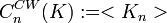$C_n^{CW}(K):=$

and the boundary maps via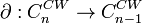$\partial:C_n^{CW}\rightarrow C^{CW}_{n-1}$ by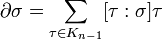$\partial\sigma=\sum_{\tau\in K_{n-1}}[\tau:\sigma]\tau$

where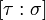$[\tau:\sigma]$ is roughly the number of times that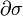$\partial\sigma$ covers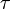$\tau$.

Let's make this precise.

For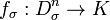$f_{\sigma}:D_{\sigma}^n\rightarrow K$ (not quite an embedding) this restricts to a map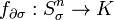$f_{\partial\sigma}:S_{\sigma}^n\rightarrow K$. Given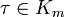$\tau\in K_m$ let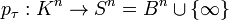$p_{\tau}:K^n\rightarrow S^n = B^n\cup\{\infty\}$ such that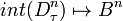$int(D^n_{\tau})\mapsto B^n$ and the rest maps to the point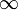$\infty$.

Example:

If you just have the segment consisting of two endpoints and the line connecting them, and call this$\tau$, then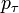$p_{\tau}$ takes the two end points to$\infty$ and the rest (the open interval) gets mapped to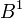$B^1$. Hence, we get a circle.

We thus can now formally define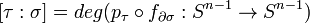$[\tau:\sigma]= deg(p_{\tau}\circ f_{\partial\sigma}:S^{n-1}\rightarrow S^{n-1})$

Theorem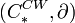$(C^{CW}_*,\partial)$ is a chain complex;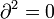$\partial^2 = 0$ and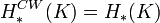$H_*^{CW}(K) = H_*(K)$

Examples:

1)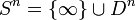$S^n = \{\infty\}\cup D^n$ for n>1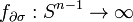$f_{\partial\sigma}: S^{n-1}\rightarrow \infty$

Hence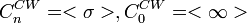$C^{CW}_n = <\sigma>, C^{CW}_0 = <\infty>$ and all the rest are zero. Hence,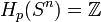$H_p(S^n) = \mathbb{Z}$ for p = 0 or n and is zero otherwise.

2) Consider the torus thought of as a square with the usual identifications and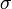$\sigma$ is the interior. Hence,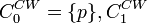$C^{CW}_0 =\{p\}, C^{CW}_1$ is generated by the figure 8 with one loop labeled a and the other labeled b, and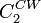$C^{CW}_2$ is generated by the entire torus.

Ie we get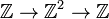$\mathbb{Z}\rightarrow\mathbb{Z}^2\rightarrow\mathbb{Z}$

Now,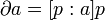$\partial a = [p:a]p$

but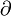$\partial$ a takes the two endpoints of a (both p) and maps them to p. Neither point is mapped to$\infty$. Hence,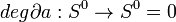$deg\partial a:S^0\rightarrow S^0 = 0$

Note: This ought to be checked from the definition of degree but was just stated in class

Now,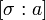$[\sigma:a]$ = the degree of the map that takes the square to the figure 8...and hence is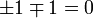$\pm 1\mp 1 = 0$.

Hence the boundary map is zero at all places, so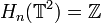$H_n(\mathbb{T}^2) = \mathbb{Z}$ if n = 0 or 2,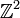$\mathbb{Z}^2$ if n = 1 and is zero otherwise.

3) Consider the Klein bottle thought of as a square with the usual identifications. Under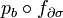$p_b\circ f_{\partial\sigma}$ takes this to a circle with side labled b.

I.e.,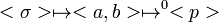$<\sigma>\mapsto\mapsto^0

$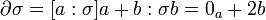$\partial\sigma = [a:\sigma]a+b:\sigma b = 0_a + 2b$

Where the sign may be negative. Or more eloquantly put: "2b or -2b, that is the question"

The kernal of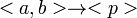$\rightarrow

$

is everything, so the homology is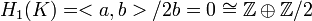$H_1(K) = /2b=0\cong\mathbb{Z}\oplus\mathbb{Z}/2$,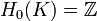$H_0(K)=\mathbb{Z}$ and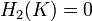$H_2(K) = 0$.

4)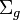$\Sigma_g$ the "g holed torus" or "surface of genus g" is formed by the normal diagram with edges identified in sets of 4 such as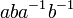$aba^{-1}b^{-1}$

So, get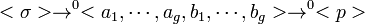$<\sigma>\rightarrow^0\rightarrow^0

$

Therefore,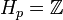$H_p = \mathbb{Z}$ if p = 2 or 0,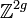$\mathbb{Z}^{2g}$ if p = 1 and zero elsewhere.

5)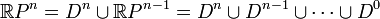$\mathbb{R}P^n= D^n\cup \mathbb{R}P^{n-1} = D^n\cup D^{n-1}\cup\cdots\cup D^0$

So,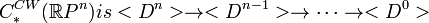$C_*^{CW}(\mathbb{R}P^n) is \rightarrow\rightarrow\cdots\rightarrow $

The boundary map alternates between 0 and 2 where it is 2 for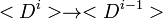$\rightarrow$ if i is even and 0 if it is odd.

Hence the homology alternates between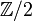$\mathbb{Z}/2$ for odd p and 0 for even p.

### Second Hour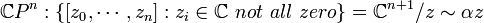$\mathbb{C}P^n:\{[z_0,\cdots,z_n]:z_i\in\mathbb{C}\ not\ all\ zero\}=\mathbb{C}^{n+1}/z\sim\alpha z$ for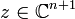$z\in\mathbb{C}^{n+1}$,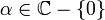$\alpha\in\mathbb{C}-\{0\}$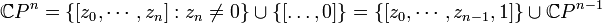$\mathbb{C}P^n=\{[z_0,\cdots,z_n]:z_n\neq 0\}\cup\{[\ldots,0]\} = \{[z_0,\cdots,z_{n-1},1]\}\cup\mathbb{C}P^{n-1}$ =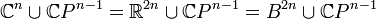$\mathbb{C}^n\cup\mathbb{C}P^{n-1} = \mathbb{R}^{2n}\cup\mathbb{C}P^{n-1} = B^{2n}\cup\mathbb{C}P^{n-1}$

I.e.,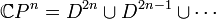$\mathbb{C}P^n = D^{2n}\cup D^{2n-1}\cup\cdots$

So,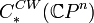$C^{CW}_*(\mathbb{C}P^n)$ alternates between 0 (for odd p) and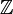$\mathbb{Z}$ for even p and thus as this also as the homology. (and clearly trivial greater than p)